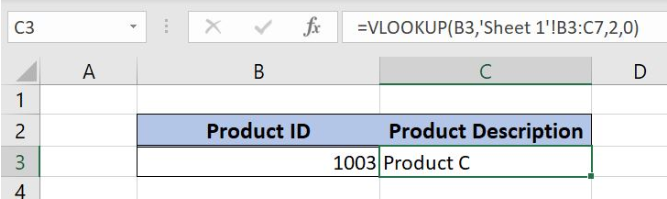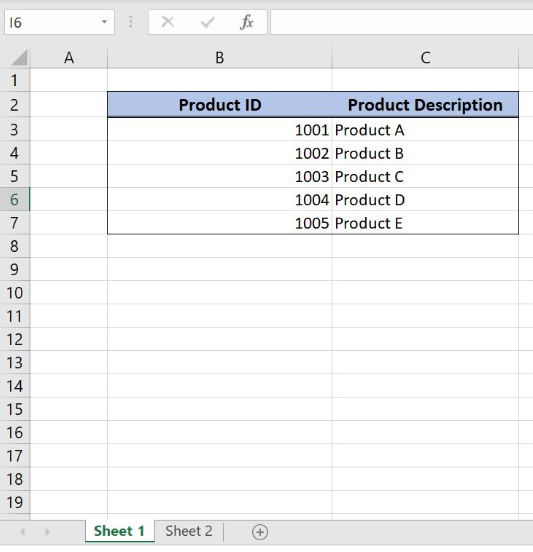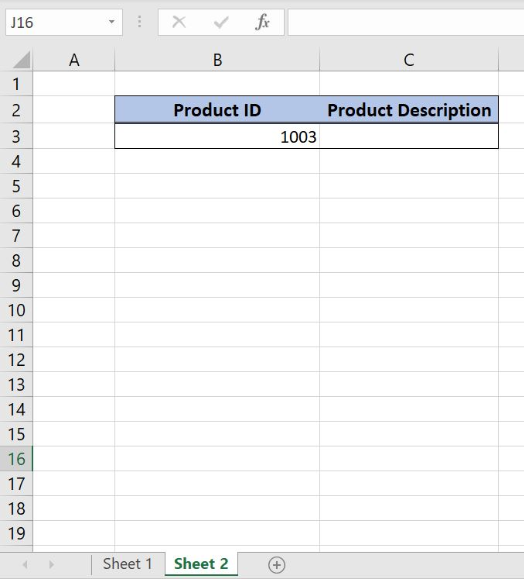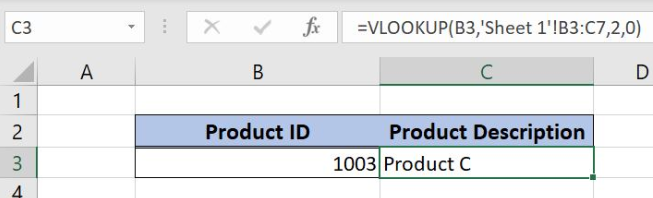Get instant live expert help with Excel or Google Sheets“My Excelchat expert helped me in less than 20 minutes, saving me what would have been 5 hours of work!”

#### Post your problem and you’ll get expert help in seconds.

Your message must be at least 40 characters
Our professional experts are available now. Your privacy is guaranteed.

# Here is How You Can Lookup Values Across Multiple Sheets in Excel

Excel allows a user to lookup values across multiple sheets using the VLOOKUP function. This step by step tutorial will assist all levels of Excel users to lookup values across multiple sheets in ExcelFigure 1. The result of the VLOOKUP function

## Syntax of the VLOOKUP Formula

The generic formula for the VLOOKUP function is:

`=VLOOKUP(lookup_value, table_array, col_index_num, range_lookup)`

The parameters of the VLOOKUP function are:

• lookup_value – a value that we want to find in a table_array
• table_array – a range in which we want to lookup
• col_index_num – a column number in table_array from which we would like to get a value
• range_lookup – default value 0. This means that we want to find an exact match for a lookup value.

## Setting up Our Data for the VLOOKUP Function

Let’s look at the structure of the data that we will use. In the range B2:C7 of Sheet 1, we have a table from which we want to pull data, while in the cell F3 of Sheet 2 we want to get a value. Tables consist of the columns “Product ID” and “Product Description”.Figure 2. The data table in Sheet 1 that we will use in the VLOOKUP exampleFigure 3. Sheet 2 where we want to get a result

Get the Product Description from Another Sheet Using the VLOOKUP Function

Our goal is to obtain the “Product Description” from the Sheet 1 and populate it in Sheet 2, into the cell C3.

The formula looks like:

`=VLOOKUP(B3, 'Sheet 1'!B3:C7, 2, 0)`

In our example, the lookup_value is the B3 cell in “Product ID” column of Sheet 2. The parameter table_array is ‘Sheet 1’!B3:C7 of Sheet 1 because we want to find value from the range B3:B7. When we reference the sheet we have to put the name of the Worksheet under single quotations and exclamation mark. Col_index_num has value 2, as we want to pull value from the second column of the range. Finally, range_lookup has value 0, because we want to find an exact match of “Product ID” values.

To apply the VLOOKUP function, we need to follow these steps:

• Select cell C3 and click on it
• Insert the formula: `=VLOOKUP(B3, 'Sheet 1'!B3:C7, 2, 0)`
• Press enter.Figure 4. Using the VLOOKUP function

As a result, we will get Product C in the cell C3 of Sheet 2. As you can see, the value of “Product Description” in C3 in Sheet 1 table is Product C. The function pulls this value and returns it to the cell C3 as a result.

Most of the time, the problem you will need to solve will be more complex than a simple application of a formula or function. If you want to save hours of research and frustration, try our live Excelchat service! Our Excel Experts are available 24/7 to answer any Excel question you may have. We guarantee a connection within 30 seconds and a customized solution within 20 minutes.

Solution examplesI have a pivot table in which per order (on the rows) stands how much products they ordered per size (on the columns). I want to determine which combinations of quantities of sizes people order. And I want to count these combinations.
Solved by E. W. in 60 minsI have 500 numbers in column A with aproximatly 15digits. In column B I have 5 numbers with 6 digits Can a find a formula wich can transfer all numbers from A column wich start with some 6 digitsa from B column
Solved by V. L. in 32 minsI have a sheet with 3 columns. First column is a code for records in column B (has 563 records). What I need is a formula to show me if what is in column C (has 4400 records) is in column B and if it is to take the code for that record. Example: Column A(code corresponding to column B): 12, 14, 15, 19 Column B(names): asd, adf, ade, aqw Column C(names): akd, adf, ade, anb, wgs I need something that would say, if record in column C (for example adf) is matching record in column B (adf) return the code from column A (the code corresponding to that record in column B),if not return blank.
Solved by C. J. in 8 minsIf the data in A matches the data in C, then I need the data in E to be placed in B
Solved by X. W. in 40 minsI have a workbook with 2 sheets. First sheet has column A which is a device name, then columns B - infinity that have numeric "tags" for that device. Each device can have one or 20 "tags", tags are all numerical. Worksheet 2 has column A which is numeric value and B which is a human readable value of what the tag is. Question is, how do I replace all the tag numbers in worksheet 1 with the actual human readable values is sheet 2?
Solved by T. H. in 16 mins